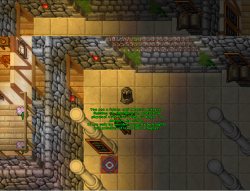# (OTXSERVER TFS1.2 BASSED)Look HP/Mana regeneration and percent on look

#### Liikuid

##### Spin Machine!
(Title too redundant lol)
Hello mates, this is my first post for C++ section. I saw a lot of post about "i want to see those attributes like health regeneration when i look" Then i've decided to explore about how to do, and works well. Here we go:

Item.cpp

above
C++:
``````            if (it.abilities->speed) {
if (begin) {
begin = false;
s << " (";
} else {
s << ", ";
}

s << "speed " << std::showpos << (it.abilities->speed >> 1) << std::noshowpos;
}``````

C++:
``````if (it.abilities->regeneration) {
if (begin) {
begin = false;
s << " (";
}
else {
s << ", ";
}

s << "+" << std::showpos << it.abilities->healthGain << std::noshowpos << " hp/s";
}
if (it.abilities->regeneration) {
if (begin) {
begin = false;
s << " (";
}
else {
s << ", ";
}

s << "+" << std::showpos << it.abilities->manaGain << std::noshowpos << " mana/s";
}
if (it.abilities->statsPercent[STAT_MAXHITPOINTS]) {
if (begin) {
begin = false;
s << " (";
}
else {
s << ", ";
}

s << "hp " << std::showpos << it.abilities->statsPercent[STAT_MAXHITPOINTS] - 100 << std::noshowpos << "%";
}
if (it.abilities->statsPercent[STAT_MAXMANAPOINTS]) {
if (begin) {
begin = false;
s << " (";
}
else {
s << ", ";
}

s << "mana " << std::showpos << it.abilities->statsPercent[STAT_MAXMANAPOINTS] - 100 << std::noshowpos << "%";
}``````
Compile and test. This is the code and it works well, as I said at the beginning of this post.

Look:Thanks

Last edited:
•115820, pepsiman and Deatthraz

#### 115820

##### New Member
(Title too redundant lol)
Hello mates, this is my first post for C++ section. I saw a lot of post about "i want to see those attributes like health regeneration when i look" Then i've decided to explore about how to do, and works well. Here we go:

Item.cpp

above
C++:
``````            if (it.abilities->speed) {
if (begin) {
begin = false;
s << " (";
} else {
s << ", ";
}

s << "speed " << std::showpos << (it.abilities->speed >> 1) << std::noshowpos;
}``````

C++:
``````if (it.abilities->regeneration) {
if (begin) {
begin = false;
s << " (";
}
else {
s << ", ";
}

s << "+" << std::showpos << it.abilities->healthGain << std::noshowpos << " hp/s";
}
if (it.abilities->regeneration) {
if (begin) {
begin = false;
s << " (";
}
else {
s << ", ";
}

s << "+" << std::showpos << it.abilities->manaGain << std::noshowpos << " mana/s";
}
if (it.abilities->statsPercent[STAT_MAXHITPOINTS]) {
if (begin) {
begin = false;
s << " (";
}
else {
s << ", ";
}

s << "hp " << std::showpos << it.abilities->statsPercent[STAT_MAXHITPOINTS] - 100 << std::noshowpos << "%";
}
if (it.abilities->statsPercent[STAT_MAXMANAPOINTS]) {
if (begin) {
begin = false;
s << " (";
}
else {
s << ", ";
}

s << "mana " << std::showpos << it.abilities->statsPercent[STAT_MAXMANAPOINTS] - 100 << std::noshowpos << "%";
}``````
Compile and test. This is the code and it works well, as I said at the beginning of this post.

Look:
View attachment 36504

Work for me